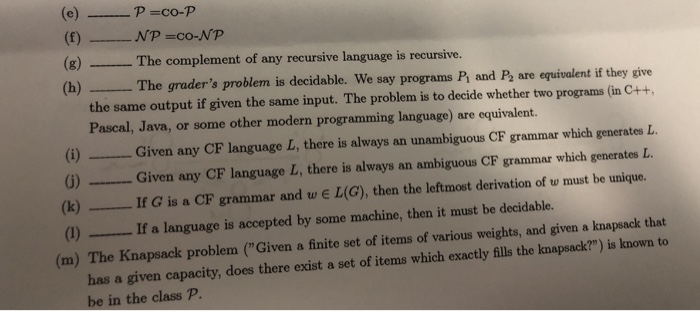Question
true or False with prove?e) true,

Given a polynomial-time TM M for L, we can modify M to accept the complement of L as follows:

1)Make each accepting state of M a nonaccepting state from which there are no moves. Thus, if M accepts, the new TM (L) will halt without accepting.

2)Create a new state q, which is the only accepting state in the new TM. For each state-symbol combination that has no move, the new TM enters state q, whereupon it accepts and halts.

f) Theorem NP=co-NP if and only if the complement of some NP-complete problem is in NP.

Proof

1)(Only if): Should NP and Co-NP be the same

¨Every NP-complete problem L, being in NP, is also in co-NP

¨The complement of a problem in Co-NP is in NP è the complement of L is in NP

2)(If)

¨Let P be an NP-complete problem

¨Suppose Pc is in NP

¨For each L in NP, there is a polynomial-time reduction of L to P

¨Lc is polynomial reducible to Pc

_è Lc is in NP

g) false ,

RE languages are not closed under complement on which means complement of RE language need not be RE.

h) Decidability in terms of a Turing machine, a problem is said to be a Decidable problem if there exist a corresponding Turing machine which halts on every input with an answer- yes or no. It is also important to know that these problems are termed as turing decidable since a Turing machine always halts on every input, accepting or rejecting it.

i) false,

If a context free grammar G is ambiguous, language generated by grammar L(G) may or may not be ambiguous.

j) false,

An ambiguous grammar is a context free grammar for which there exists a string that can have more than one leftmost derivation or parse trees. Unambiguous grammar is a context free grammar for which every valid string has a unique leftmost derivation or parse tree.

k) true,

In ambiguous grammar, a string can have two or more leftmost derivations but, in unambiguous grammar, a string has a unique leftmost derivation.

l) true,

A language is Decidable iff there is a Turing Machine which will accept strings in the language and reject strings not in the language.

m) false

Knapsack problem is NP-completeNP-complete when the numbers are given as binary numbers. In this case, the dynamic programming will take exponentially many steps (in the size of the input, i.e. the number of bits in the input) to finish

#### Earn Coins

Coins can be redeemed for fabulous gifts.

Similar Homework Help Questions
• ### If L1 and L2 are Regular Languages, then L1  ∪ L2  is a CFL. Group of answer choices...

If L1 and L2 are Regular Languages, then L1  ∪ L2  is a CFL. Group of answer choices True False Flag this Question Question 61 pts If L1 and L2 are CFLs, then L1  ∩ L2 and L1 ∪ L2 are CFLs. Group of answer choices True False Flag this Question Question 71 pts The regular expression ((ac*)a*)* = ((aa*)c*)*. Group of answer choices True False Flag this Question Question 81 pts Some context free languages are regular. Group of answer choices True...